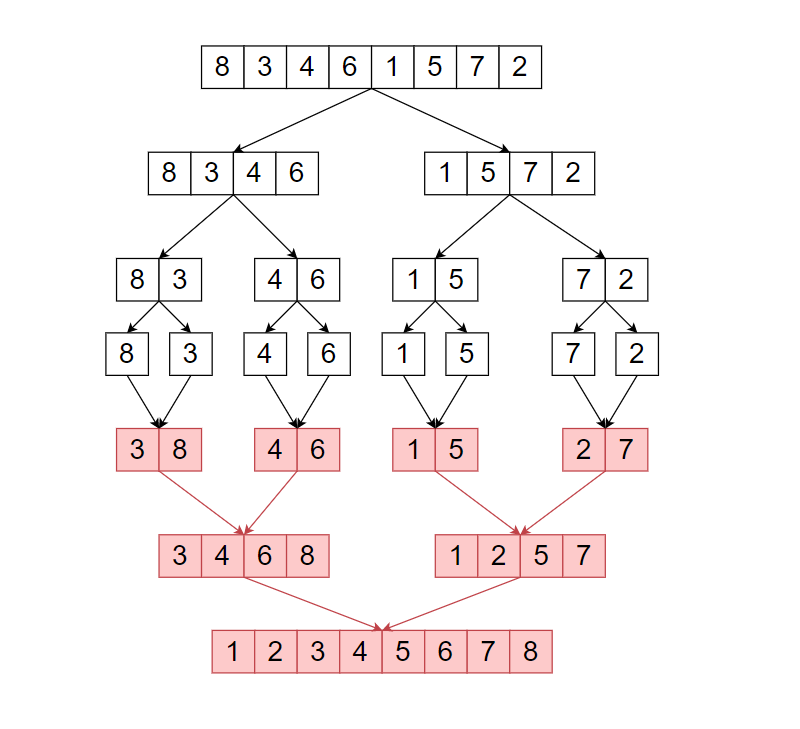Problem title
Difficulty
Avg time to solve

Multiply Strings
Moderate
35 mins
Quick Sort
Moderate
10 mins
Copy And Reverse The Array
Easy
10 mins
Compare Version Numbers
Easy
10 mins
Roots of the tree having minimum height
Moderate
25 mins
Occurrence of X in a Sorted Array
Easy
26 mins
Non-Decreasing Array
Moderate
35 mins
Binary Tree Pruning
Moderate
20 mins
Number Pattern 1
Easy
10 mins
Sum of even & odd
Easy
15 mins20

# Merge Sort

Difficulty: EASY
Avg. time to solve
15 min
Success Rate
85%

Problem Statement

#### Example :

``````Merge Sort Algorithm -

Merge sort is a Divide and Conquer based Algorithm. It divides the input array into two-parts, until the size of the input array is not ‘1’. In the return part, it will merge two sorted arrays a return a whole merged sorted array.
````````````The above illustrates shows how merge sort works.
``````
##### Note :
``````It is compulsory to use the ‘Merge Sort’ algorithm.
``````
##### Input format :
``````The first line of input contains an integer ‘T’ denoting the number of test cases.
The next 2*'T' lines represent the ‘T’ test cases.

The first line of each test case contains an integer ‘N’ which denotes the size of ‘ARR’.

The second line of each test case contains ‘N’ space-separated elements of ‘ARR’.
``````
##### Output Format :
``````For each test case, print the numbers in non-descending order
``````
##### Note:
``````You are not required to print the expected output; it has already been taken care of. Just implement the function.
``````
##### Constraints :
``````1 <= T <= 50
1 <= N <= 10^4
-10^9 <= arr[i] <= 10^9

Time Limit : 1 sec
``````
##### Sample Input 1 :
``````2
7
3 4 1 6 2 5 7
4
4 3 1 2
``````
##### Sample Output 1 :
``````1 2 3 4 5 6 7
1 2 3 4
``````
##### Explanation For Sample Input 1:
``````Test Case 1 :

Given ‘ARR’ : { 3, 4, 1, 6, 2, 5, 7 }

Then sorted 'ARR' in non-descending order will be : { 1, 2, 3, 4, 5, 6, 7 }. Non-descending order means every element must be greater than or equal to the previse element.

Test Case 2 :

Given ‘ARR’ : { 4, 3, 1, 2 }

Then sorted 'ARR' in non-descending order will be : { 1, 2, 3, 4 }.
``````
##### Sample Input 2 :
``````2
4
5 4 6 7
3
2 1 1
``````
##### Sample Output 2 :
``````4 5 6 7
1 1 2
``````Console